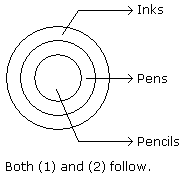# Verbal Reasoning - Syllogism

Exercise : Syllogism - Syllogism 1
Directions to Solve

In each of the following questions two statements are given and these statements are followed by two conclusions numbered (1) and (2). You have to take the given two statements to be true even if they seem to be at variance from commonly known facts. Read the conclusions and then decide which of the given conclusions logically follows from the two given statements, disregarding commonly known facts.

• (A) If only (1) conclusion follows
• (B) If only (2) conclusion follows
• (C) If either (1) or (2) follows
• (D) If neither (1) nor (2) follows and
• (E) If both (1) and (2) follow.

6.

Statements: All the actors are girls. All the girls are beautiful.

Conclusions:

1. All the actors are beautiful.
2. Some girls are actors.

Only (1) conclusion follows
Only (2) conclusion follows
Either (1) or (2) follows
Neither (1) nor (2) follows
Both (1) and (2) follow
Explanation: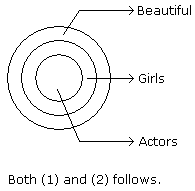7.

Statements: All the windows are doors. No door is a wall.

Conclusions:

1. Some windows are walls.
2. No wall is a door.

Only (1) conclusion follows
Only (2) conclusion follows
Either (1) or (2) follows
Neither (1) nor (2) follows
Both (1) and (2) follow
Explanation: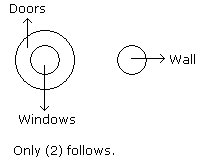8.

Statements: All cups are books. All books are shirts.

Conclusions:

1. Some cups are not shirts.
2. Some shirts are cups.

Only (1) conclusion follows
Only (2) conclusion follows
Either (1) or (2) follows
Neither (1) nor (2) follows
Both (1) and (2) follow
Explanation: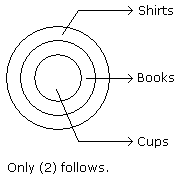9.

Statements: Some cows are crows. Some crows are elephants.

Conclusions:

1. Some cows are elephants.
2. All crows are elephants.

Only (1) conclusion follows
Only (2) conclusion follows
Either (1) or (2) follows
Neither (1) nor (2) follows
Both (1) and (2) follow
Explanation: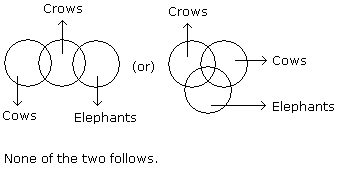10.

Statements: All the pencils are pens. All the pens are inks.

Conclusions:

1. All the pencils are inks.
2. Some inks are pencils.

Only (1) conclusion follows
Only (2) conclusion follows
Either (1) or (2) follows
Neither (1) nor (2) follows
Both (1) and (2) follow